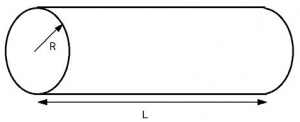# Water Volume Calculation Formula of a Cylinder

Knowing how much water your tanks hold is very important in aquaponics. Here is the formula for calculating water volume in a cylinder tank.### How to Calculate the Volume of Water in a Rectangle

There are many commercial grow tanks or even homemade welded cylinders that you might have access to, however we are going to use the measurements of a 55 gallon barrel to demonstrate. I default to these numbers to show you the example, but you can enter any numbers you want for your specifics.

### Dimensions of 2 types of 55 Gallon Barrels

There are 2 types of 55 gallon barrels, feel free to try both measurements.

55 Gallon Open Barrel
The open barrels are a bit higher and skinnier and measure 23.8W x 37.7H (inches) outside diameter.

55 Gallon Closed Barrel
The closed barrels measure 23.3W x 34.9H (inches) outside diamter.

### The Formula for Volume of a Cylinder

The formula for volume of a cylinder is Π * Radius ² * Height.

### Estimation of Inside Measurements

To make things more accurate, neither of these cylinders are true cylinders, but more like ovals (subtract 1.25 inches). And we need to account for the size of the walls (subtract half inch from top and sides) and none of the barrels are actually filled to the very brim and the height includes the upper support ring which does not hold water (minus 3 inches).

So, the estimate using these numbers on a 55 gallon barrel drum with modified dimensions of 23.8 -1.25 -.5 = 22.05 (11.025 radius), and 37.7 -3 -.5 = height of 34.2 inches

I have seen grow beds an tanks made from a welded sheet of aluminum then lined and painted on the inside to make a perfect cylinder. This calculation would be perfect for that type of tank!

### Cylinder Volume Calculator

 First, we need to get the cubic inches (Radius is half of diameter): Inside Radius (inches) Inside Height (inches) Cubic Inches Now we convert to cubic feet (cubic inches x 0.000578704) Cubic Feet To calculate gallons, we multiply cubic feet by 7.47: Gallons (Water only) To figure out how much this weighs (and if your subfloor can support the weight), we multiply 8.34 (freshwater weight of 1 gallon) x how many gallons: Weight (only the weight of the water) Note that if you are using this in grow beds, you will likely only get half or less of the water you plan for into the grow bed, the rest is filled with media (like gravel or hydroton).

Previous post:

Next post: